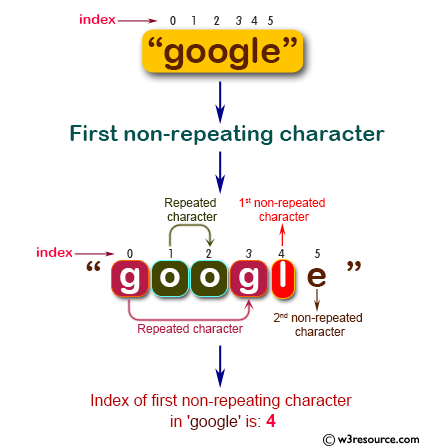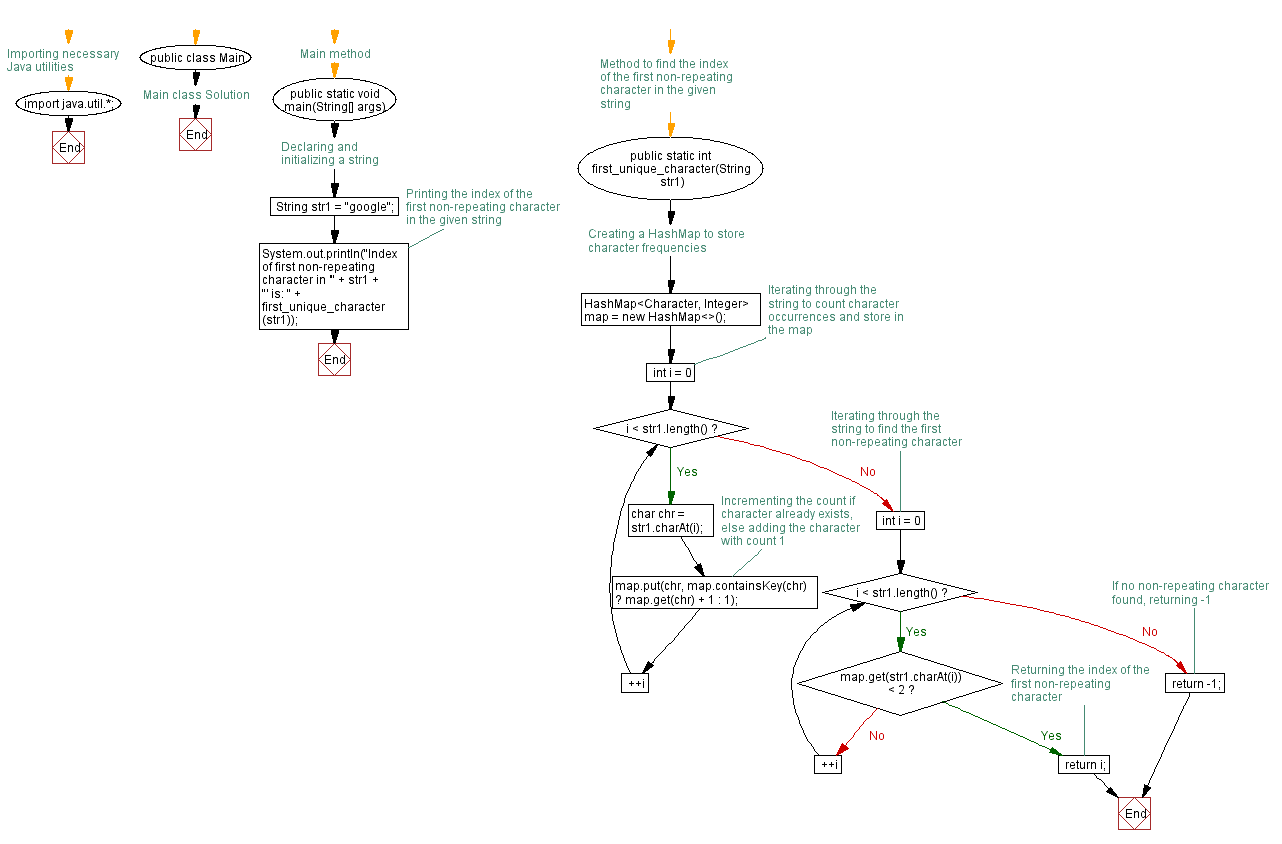﻿ Java programming exercises: Find the index of first non-repeating character in a given string - w3resource# Java Exercises: Find the index of first non-repeating character in a given string

## Java Basic: Exercise-187 with Solution

Write a Java program to find the index of first non-repeating character in a given string.

Pictorial Presentation:Sample Solution:

Java Code:

``````import java.util.*;
public class Solution {
public static void main(String[] args) {
System.out.println("Index of first non-repeating character in '" + str1 + "' is: " + first_unique_character(str1));
}

public static int first_unique_character(String str1) {
HashMap < Character, Integer > map = new HashMap < > ();
for (int i = 0; i < str1.length(); ++i) {
char chr = str1.charAt(i);
map.put(chr, map.containsKey(chr) ? map.get(chr) + 1 : 1);
}
for (int i = 0; i < str1.length(); ++i) {
if (map.get(str1.charAt(i)) < 2) {
return i;
}
}
return -1;
}
}
```
```

Sample Output:

```Index of first non-repeating character in 'google' is: 4
```

Flowchart:Java Code Editor:

Company:

What is the difficulty level of this exercise?

﻿

## Java: Tips of the Day

Parsing dates:

```import java.io.*;
import java.util.*;
import java.text.*;

String s = "2001/09/23 14:39";

SimpleDateFormat formatter = new SimpleDateFormat ("yyyy/MM/dd H:mm");
Date d = formatter.parse(s, new ParsePosition(0));
```